Class 10 Science: CBSE Sample Question Paper (2019-20) - 7

# Class 10 Science: CBSE Sample Question Paper (2019-20) - 7 Notes | Study CBSE Sample Papers For Class 10 - Class 10

## Document Description: Class 10 Science: CBSE Sample Question Paper (2019-20) - 7 for Class 10 2022 is part of CBSE Sample Papers For Class 10 preparation. The notes and questions for Class 10 Science: CBSE Sample Question Paper (2019-20) - 7 have been prepared according to the Class 10 exam syllabus. Information about Class 10 Science: CBSE Sample Question Paper (2019-20) - 7 covers topics like and Class 10 Science: CBSE Sample Question Paper (2019-20) - 7 Example, for Class 10 2022 Exam. Find important definitions, questions, notes, meanings, examples, exercises and tests below for Class 10 Science: CBSE Sample Question Paper (2019-20) - 7.

Introduction of Class 10 Science: CBSE Sample Question Paper (2019-20) - 7 in English is available as part of our CBSE Sample Papers For Class 10 for Class 10 & Class 10 Science: CBSE Sample Question Paper (2019-20) - 7 in Hindi for CBSE Sample Papers For Class 10 course. Download more important topics related with notes, lectures and mock test series for Class 10 Exam by signing up for free. Class 10: Class 10 Science: CBSE Sample Question Paper (2019-20) - 7 Notes | Study CBSE Sample Papers For Class 10 - Class 10
 1 Crore+ students have signed up on EduRev. Have you?

Class-X
Science-086
SAMPLE QUESTION PAPER 2019-20

TIME: 3 Hrs.
M.M: 80

General Instructions:
1. The question paper comprises three sections - A, B and C. Attempt all the sections.
2. All questions are compulsory.
3. Internal choice is given in each section.
4. All questions in Section A are one - mark questions comprising MCQ, VSA type and
assertion-reason type questions. They are to be answered in one word or in one sentence.
5. All questions in Section B are three - mark, short - answer type questions. These are to be answered in about 50 - 60 words each.
6. All questions in Section C are five - mark, long - answer type questions. These are to be answered in about 80 - 90 words each.
7. This question paper consists of a total of 30 questions.

SECTION A

Q.1. Write two advantages associated with water harvesting at the community level.    (1 Mark)
Ans:
(i) Recharge the ground water level.
(ii) Bring rivers back to life.

Q.2. How does valency of an element vary across a period?    (1 Mark)
Ans:
The valency of an element first increases and then decreases across a period.

Q.3. A ray of light AM is incident on a concave m irror as shown below. Then which of the following ray diagrams is correct for the reflected ray?    (1 Mark)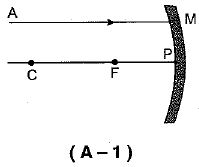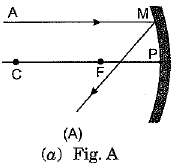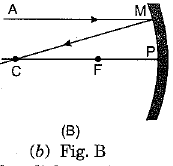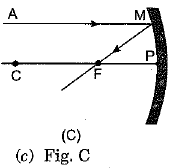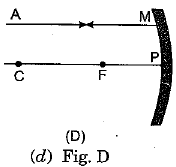Ans:
(c) As the incident light ray is coming parallel to the principal axis of given concave mirror, the reflected ray must pass through the principal focus of mirror.

Q.4. For Q.Nos. 4(i)-4(iv) are based on the information given below. Study these information related to answer the questions that follow :
The pH scale can be used to determine the strength of acid solutions as well as basic solutions by making use of hydrogen ion concentrations in them. Consider two solutions A and B having pH values 3 and 9.5, respectively.
(i) Which solution will turn blue litmus red?    (1 Mark)
(ii) Which solution will turn phenolphthalein from colourless to pink?    (1 Mark)
(iii) Which of the following substances would have pH less than 7?    (1 Mark)
(a) Vinegar
(b) Antacid
(c) Water
(d) Soap
(iv) Water is a neutral substance. What colour will you get when you add a few drops of universal indicator to a test tube containing distilled water?    (1 Mark)
(a) Green
(b) Dark red
(c) Blue
(d) Yellow
Ans:
(i) Solution A will turn bluelitmus to red as its value of pH is 3.
(ii) Solution B will turn phenolphthalein from colourless to pink as it is basic in nature.
(iii) (b) Acidic substances will have pH value less than 7.
(iv) (a) It turns the universal indicator solution green as its pH value is 7.

Q.5. Which of the following are exothermic processes ?    (1 Mark)
(i) Reaction of water with quick lime.
(ii) Dilution of an acid.
(iii) Evaporation of water.
(iv) Sublimation of camphor (crystals).
(a) (i)and(ii)
(b) (ii) and (iii)
(c) (i)and(iv)
(d) (iii)and(iv)
OR
Which of the following does not belong to the same homologous series?
(a) CH4
(b) C2H6
(c) C3H8
(d) C4H8
Ans:
(a) Exothermic reactions are those reactions in which heat is released along with the formation of products. When quick lime reacts with water, a large amount of heat is released along with the formation of calcium hydroxide. Similarly, the process of dissolving an acid or base in water is a highly exothermic reaction. Evaporation of water and sublimation of camphor are endothermic reactions.
OR
(d) A series of compounds in which the same functional group substitutes for hydrogen in a carbon chain is called as homologous series. The option (d) follows the generic formula of alkenes i.e., CnH2n, while others follow the generic formula of alkanes Le.f CnH2n+2.

Q.6. When a 4V battery is connected across an unknown resistor there is a current of 100mA in the circuit. The value of the resistance of the resistor is:    (1 Mark)
(a) 4Ω
(b) 40Ω
(c) 400Ω
(d) 0.4Ω
Ans:
(b) =40 Ω.
V=IR, V = 4V, I = 100 mA = 0.1 A
Hence R = V/l = 4/0.1 Ω = 40 Ω

Q.7. Which one of the following is the most correct sequence in respect of aerobic respiration?    (1 Mark)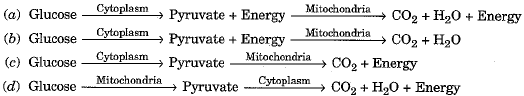Ans:
(a) In aerobic respiration pyruvate is formed in cytoplasm and is further broken down  in mitochondria. During both steps, energy is released.

Q.8. Which one of the following terms denotes the formation of two independent species due to genetic drift, geographical isolation and natural selection?    (1 Mark)
(a) Classification
(b) Reproduction
(c) Evolution
(d) Speciation
Ans:
(d) The phenomenon leading to the origin of new species from existing ones is called speciation. The important factors which could lead to the rise of new species are genetic drift, geographical isolation and natural selection.

Q.9. A light ray enters from medium A to medium B as shown in the figure. The refractive index of medium B relative to A will be:    (1 Mark)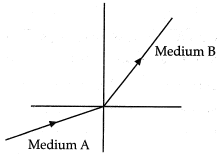(a) Greater than unity
(b) Less than unity
(c) Equal to unity
(d) Zero
Ans:
(a) Since, light rays in medium B bends towards normal. So, it has greater refractive index and lesser velocity of light with respect to medium A. So, refractive index of medium B with respect to medium A is greater than unity.

Q.10. Which of the following reactions is an endothermic reaction?
(i) Burning of coal
(ii) Decomposition of vegetable matter into compost
(iii) Process of respiration.
(iv) Decomposition of calcium carbonate to form quick lime and carbon dioxide.    (1 Mark)
Ans:
(iv) Decomposition of calcium carbonate to form quick lime and carbon dioxide.

Q.11. A triple bond is represented by writing a single bond____________ times.    (1 Mark)
Ans:
three

Q.12. When the ciliary muscles are relaxed, focal length of eye lens is    (1 Mark)
(a) maximum
(b) minimum
(c) Neither maximum nor minimum
(d) equal to least distance of distinct vision
Ans:
(a) When we are looking at distant objects, the ciliary muscles are relaxed and the eye lens becomes thin, Consequently, the focal length of the eye lens becomes maximum.

Q.13. For question numbers 13 and 14, two statements are given- one labelled Assertion (A) and the other labelled Reason (R). Select the correct answer to these questions from the codes (i), (ii), (iii) and (iv) as given below:
(i) Both A and R are true and R is correct explanation of the assertion.
(ii) Both A and R are true but R is not the correct explanation of the assertion.
(iii) A is true but R is false.
(iv) A is false but R is true.
Assertion (A): Humans are not truly aerobic.
Reason (R): They produce lactic acid anaerobically.
OR
Assertion (A) : In human male, testes are extra-abdominal which are present inside scrotum
Reason (R) : Scrotum has a relatively lower temperature needed for the production and storage of sperms.    (1 Mark)
Ans:
(b) Human are aerobically respiring animals, but sometimes anaerobic respiration takes place in certain tissues like skeletal muscles, which do not get immediately as much oxygen as it requires. Therefore, the muscles respire anaerobically and produce lactic acid from glucose.
Or
(a) Formation of sperms needs lower temperature than the normal body temperature. Hence, testes lie outside the body cavity in the scrotum.

Q.14. For question numbers 13 and 14, two statements are given- one labelled Assertion (A) and the other labelled Reason (R). Select the correct answer to these questions from the codes (i), (ii), (iii) and (iv) as given below :
(i) Both A and R are true and R is correct explanation of the assertion.
(ii) Both A and R are true but R is not the correct explanation of the assertion.
(iii) A is true but R is false.
(iv) A is false but R is true.
Assertion : A fuse wire is always connected in parallel with the mainline.
Reason : If a current larger than the specified value flows through the circuit, fuse wire melts.    (1 Mark)
Ans:
(iv) Assertion is false but reason is true.

SECTION B

Q.15. 2 g of silver chloride is taken in a china dish and the china dish is placed in sunlight for sometime. What will be your observation in this case? Write the chemical reaction involved in the form of a balanced chemical equation. Identify the type of chemical reaction.
OR
Identify the type of reactions taking place in each of the following cases and write the balanced chemical equation for the reactions.
(a) Zinc reacts with silver nitrate to produce zinc nitrate and silver.
(b) Potassium iodide reacts with lead nitrate to produce potassium nitrate and lead iodide.    (3 Mark)
Ans: White silver chloride turns grey in Sunlight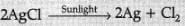Decomposition reaction/photolytic decompositionOr
(a) Displacement reaction
Zn(s) + 2AgNO3(aq) → Zn(NO3)2(aq)+ 2Ag(s)
(b) Double displacement reaction
2KI(aq) + Pb(NO3)2(aq) → 2KNO3(aq) + PbI2(s)
Deduct 1/2 mark for non-balanced equation.

Q.16. A compound 'X' of sodium is used as an antacid and it decomposes on strong heating.
(i) Name the compound 'X'and give its chemical formula.
(ii) Write a balanced chemical equation to represent the decomposition of 'X'.
(iii) Give one use of compound 'X' besides an antacid.
Or
You are provided with 90 mL of distilled water and 10 mL of concentrated sulphuric acid to prepare dilute sulphuric acid.
(i) What is the correct way of preparing dilute sulphuric acid? Give reason.
(ii) How will the concentration of H3O+ ions change on dilution?    (3 Mark)
Ans:
(i) Sodium bicarbonate/Sodium hydrogencarbonate/ baking soda and its formula is NaHCO3
(ii)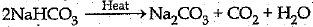(iii) It is used in fire extinguisher and for baking, (any one)
Or
(i) Add 10 mL of concentrated sulphuric acid slowly to 90 mL of water with constant stirring. Dilution of acid is a highly exothermic process. If water is added to concentrated sulphuric acid, heat generated causes the mixture to splash leading to burns and the glass container can break.
(ii) Decreases per unit volume.

Q.17. 2g of silver chloride is taken in a china dish and the china dish is placed in sunlight for sometime. What will be your observation in this case? Write the chemical reaction involved in the form of a balanced chemical equation. Identify the type of chemical reaction.
Or
Identify the type of reactions taking place in each of the following cases and write the balanced chemical equation for the reactions.
(a) Zinc reacts with silver nitrate to produce zinc nitrate and silver.
(b) Potassium iodide reacts with lead nitrate to produce potassium nitrate and lead iodide.    (3 Mark)
Ans:
White silver chloride taken in the china dish turns grey in sunlight.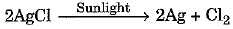It is a photolytic decomposition reaction.
Or
(a) This is a displacement reaction.
The balanced chemical equation is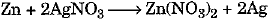(b) This is a double displacement reaction.
The balanced chemical equation is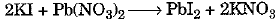Q.18. Two identical bulbs B1 and B2; and am m eter A1 and A2 are connected as shown in the figure below: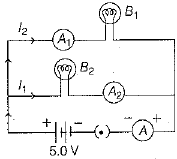When both bulbs glow, then current of 2A is recorded by ammeter A. Then, what happened to the glow of B2 bulb, if B1 gets fused?    (3 Mark)
Ans:
Resistance of the combination of two bulbs in parallel is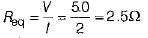if R is the resistance of each wire, then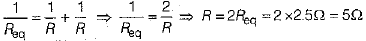∴ Current in each bulb,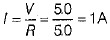Thus, when bulb B1 gets fused,Then the current in B2 remains same as I1 = I2 = 1A . So, its glow remains unaffected.

Q.19. List three techniques that have been developed to prevent pregnancy. Which one of these techniques is not meant for males? How does the use of these techniques have a direct impact on the health and prosperity of a family?    (3 Mark)
Ans:
Techniques to prevent pregnancy are:
(i) Mechanical barriers like cervical cap, condoms etc.
(ii) Surgical methods like tubectomy, vasectomy.
(iii) Using IUCD's like loop, Copper-T.
IUCD's (like loop, Copper-T) and also oral contraceptives are not meant for males.
Impact of contraceptives on health and prosperity of family are:

(i) Better standard of living.
(ii) Better and improved resources.
(iii) More focus on children who have already born.
(iv) Prevention from STDs like HIV-AIDS, syphilis etc.

Q.20. In a pea plant the trait of flowers bearing purple colour (PP) is dominant over white colour (pp). Explain the inheritance pattern of F1 and F2 generations with the help of a cross following the rules of inheritance of traits. State the visible characters of F1 tand F2 progenies.    (3 Mark)
Ans:
Let purple trait be represented by: PP White trait be : pp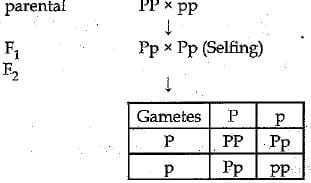Visible characters of Fprogeny all flowers are purple coloured and in F2 progenies 3 are purple coloured and 1 is white coloured flower.

Q.21. Name the plant Mendel used for his experiment. What type of progeny was obtained by Mendel in F1 and F2 generations when he crossed the tall and short plants? Write the ratio he obtained in F2 generation plants.
Or
List two differences between acquired traits and inherited traits by giving an example of each.    (3 Mark)
Ans:
Mendel used Garden Pea for his experiments.
Organisms used by Mendel for his experiments was pea plant. Mendel took a pure tall plant (TT) and crossed it with a pure dwarf (tt) plant. The progeny thus obtained was called F1 progeny (First filial progeny).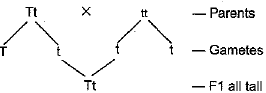Then he self pollinated the F1 progeny to obtain F2 generation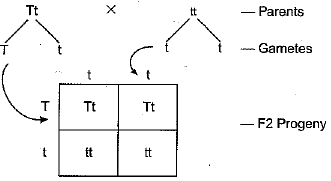In F2 generation he found that 75% plants were tall and 25% were dwarf.
ITT : 2Tt: ltt
Thus, it is seen that he got all tall in FI and both Tall and short in F2.
Or
The differences between acquired traits and inherited traits are :

 Acquired traits Inherited traits (i) An organism acquires during its life time. (i) An organism inherits from its predecessors. (ii) Not present in the genetic makeup of an individual. (ii) Present in the genetic make up. (iii) Not inheritable. (iii) Inheritable. (iv) Change in DNA will not result in any change in such traits. (iv) Change in DNA will bring about change in such traits. Example : Body weight Example : Blood group.

Q.22. A convergent lens of power 5D is combined with a divergent lens of power-4D. Then, find the focal length and power of combination of both lenses.
Or
A concave mirror forms a real image of an object at a distance of 30 cm from the mirror, when it is kept at a distance of 50 cm from the mirror. Then, find the magnification of mirror.    (3 Mark)
Ans:
Given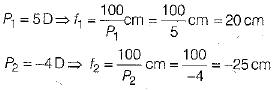If F be the focal length of combination of both lenses, then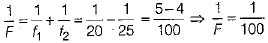∴ F = 100 cm
Power of combination of both lenses,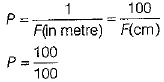∴ P = 1D
Alternate solution
Given, power of convergent lens, P1 = 5 D
Power of divergent lens, P2 = -4D
∴ Power of combination of both lenses,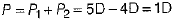∴ Focal length of combination of both lenses,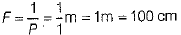Or
Given, u = -50 cm, v = -30 cm
By mirror formula,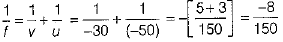∴ f = -1875 cm
∴ Magnification of the mirror,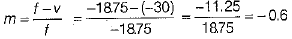Alternate solution
Given, distance of object from mirror, u = -50 cm
Distance of image from mirror, v = -30 cm  (For real image by concave mirror)
∴ Linear magnification produced by concave mirror,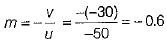Q.23. What is dam? Why do we seek to build large dams? While building large dams, which three main problems should particularly be addressed to maintain peace among local people? Mention them.    (3 Mark)
Ans:
(a) Dam is a barrier that is built across a river or a stream for storage of water,
(b) Large dam can ensure the storage of adequate water for irrigation and also for generating electricity,
(c) Social problem, economic problem and environmental problem.

Q.24. In the figure given below, a narrow beam o f white light is shown to pass through a triangular glass prism. After passing through the prism, it produces a spectrum XY on the screen.
(i) Name the phenomenon.
(ii) State the colours seen at X and Y.
(iii) Why do different colours of white light bend at different angles through a prism?    (3 Mark)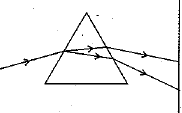Or
(i) What is visible spectrum?
(ii) Why is red used as the stopping light at traffic signals?
(iii) Two triangular glass prisms are kept together connected through their rectangular side. A light beam is passed through one side of the combination. Will there be any dispersion? Justify your answer.
Ans:
(i) The phenomenon is called dispersion.
(ii) X - Violet Y - Red
(iii) Different colours of white light bend through different angles with respect to the incident beam of light due to difference in speed of light of different wavelengths.
Or
(i) Visible spectrum is the band of coloured components of a white light beam.
(ii) Red light is scattered the least by air molecules and has longer wavelength. It travels the longest distance.
(iii) The given setup will behave like a glass slab, resulting in recombination of the seven colours to produce white light.

SECTION C

Q.25. Write the chemical formula and name of the compound which is the active ingredient of all alcoholic drinks. List its two uses. Write chemical equation and name of the product formed when this compound reacts with:
(i) sodium metal
(ii) hot concentrated sulphuric add.
OR
What is methane? Draw its electron dot structure. Name the type of bonds formed m this compound. Why are such compounds :
(i) poor conductors of electricity? and
(ii) have low melting and boiling points?
What happens when this compound burns in oxygen?    (5 Mark)
Ans: •
C2H5OH, Ethanol/Ethyl alcohol
• Good solvent; used in medicines (Any other)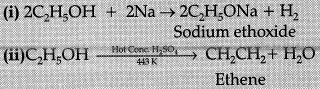Q.26. The formulae of four organic compounds are given, below: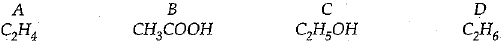(i) Which one of these compounds A, B, C or D is a saturated hydrocarbon?
(ii) Identify the organic acid and give its structural formula.
(iii) Which of the above compounds when heated at 443K in the presence o f concentrated H2SO4 forms ethene as the major product? What is the role played by concentrated H2SO4 in this reaction? Also write the chemical equation involved.

(iv) Give a chemical equation when B and C react with each other in presence of concentrated H2SO4. Name the major product formed and mention one of its important use.    (5 Mark)
Ans:
(i) D is a saturated hydrocarbon
(ii) B is an organic acid.
Structural formula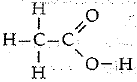(iii) C is the compound.
It acts as a dehydrating agent and removes a water molecule from ethanol.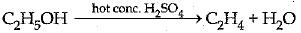(iv)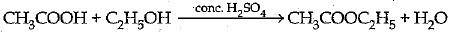Major product is Ester and it is used in rnaking perfumes / flavouring agents.

Q.27. What is a solenoid? Draw the pattern of magnetic field lines of (i) a current carrying solenoid and (ii) a bar magnet. List two distinguishing features betw een the two fields.    (5 Mark)
Ans:
A solenoid is a coil of a large number of circular turns of enamelled copper wire wrapped on a long cardboard cylindrical base. On passing electric current through a solenoid, a magnetic field is developed along the axis of solenoid.
Pattern of magnetic field lines of (i) a current carrying solenoid, and (ii) a bar magnet are shown below :
(i) Magnetic field of a current carrying solenoid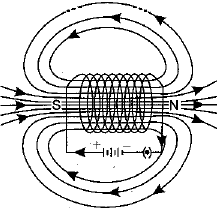(ii) Magnetic field of a bar magnet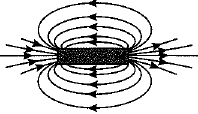Distinguishing features between the two fields are as follows :

 Field of a current carrying solenoid Field of a bar magnet 1. The field is present only when current is passed through the solenoid coil. 1. The magnetic field is a permanent field. 2. The field strength can be controlled by changing the number of turns in solenoid or current flowing through it. 2. The field strength of a bar magnet cannot be controlled.

Q.28.  A metal X is coated on iron objects to prevent rusting. Identify the metal X and write the name of the method involved in coating of X on iron. Describe the other methods to prevent iron from rusting.
Or
An iron salt A reacts with NaOH to form a green precipitate. Another iron salt B reacts with NaOH to form a brown precipitate. Identify the iron salts, A and B along with their colours and write the reactions involved.    (5 Mark)
Ans:
A thin coating of zinc is deposited on the surface of the iron object. This is done by electroplating. Since, zinc does not corrode on exposure to air, it prevents iron from rusting.
Therefore, the metal X is zinc and the method is called galvanisation.
Other methods are :
(i) By covering the surface of iron with grease, paint, varnish, enamel, etc.
(ii) By converting iron into an alloy with chromium and nickel. This alloy is called stainless steel.
(iii) By coating the surface of an iron object with chromium, tin, nickel or aluminium. These metals resist corrosion, Hence, they protect iron from rusting.
Or
(i) When a ferrous salt solution is treated with a solution of sodium hydroxide, a greenish precipitate of ferrous hydroxide is obtained. Hence, salt A is FeS04.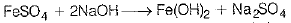(ii) When a ferric salt solution is treated with a solution of sodium hydroxide, a brown precipitate of ferric hydroxide is produced. Hence, salt B is Fe2(S04)3.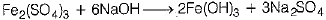(iii) The ferrous salt is generally green coloured, whereas ferric salts are brown in colour.

Q.29. A 6 cm tall object is placed perpendicular to the principal axis of a concave mirror of focal length 30 cm. The distance of the object from the mirror is 45 cm. Use mirror formula to determine the position, nature and size of the image formed. Also, draw labelled ray diagram to show the image formation in this case.
OR
An object 6 cm in size is placed at 50 cm in front of a convex lens of focal length 30 cm. At what distance from the lens should a screen be placed in order to obtain a sharp image of the object? Find the nature and size of the image. Also, draw labelled ray diagram to show the image formation in this case.    (5 Mark)
Ans:
Given :
h = 6 cm
f = - 30 cm
u = - 45 cm
Mirror formula: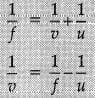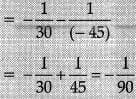v = - 90 cm from the pole of mirror
Size of the image: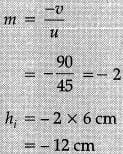Well Labelled diagram: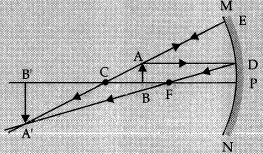Image formed will be real, inverted and enlarged.
Or
Given:
f = +30 cm
u = -50 cm
h = 6.0 cm
Lens formula: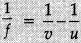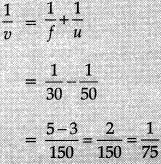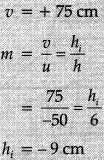Well labelled diagram: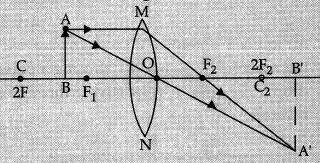Image formed is real, inverted and enlarged.

Q.30. (i) A lens produces a magnification of -0.5. Is this a converging or diverging lens? If the focal length of the lens is 6 cm, draw a ray diagram showing the image formation in this case.
(ii) A girl was playing with a thin beam of light from a laser torch by directing it from different directions on a convex lens held vertically. She was surprised to see that in a particular direction, the beam of light continues to move along the same direction after passing through the lens. State the reason for her observation. Draw a ray diagram to support your answer.
Or
(i) On entering in a medium from air, the speed of light becomes half of its value in air. Find the refractive index of that medium with respect to air?

(ii) A glass slab made of a material of refractive index n1 is kept in a medium of refractive index n2. A tight ray is incident on the slab. Draw the path of the rays of light emerging from the glass slab, if(i) n1 > n2 (ii) n1 = n2 (iii) n1< n2     (5 Mark)
Ans: (i) The image will be real and inverted, since the magnification has negative value. The lens that can produce a real and inverted image is a converging/ convex lens.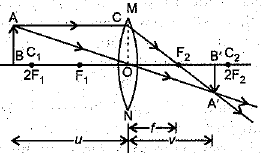In the figure OF1= OF= 6 cm.
(ii) The girl must have directed the ray of light along the direction of the optical centre of the lens because the ray of light passes straight through the optical centre of the lens.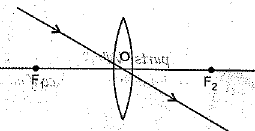Or
(i) Refractive Index of a medium (μ) = Velocity of light in vacuum / Velocity of light in the medium.
Let the velocity of light in vacuum be v1 and velocity of light in the medium be v2.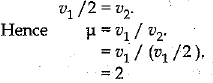(ii) (a) The ray moves towards the normal.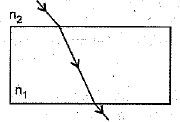(b) The ray moves undeviated.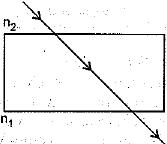(c) The ray moves away from the normal.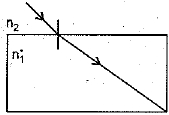The document Class 10 Science: CBSE Sample Question Paper (2019-20) - 7 Notes | Study CBSE Sample Papers For Class 10 - Class 10 is a part of the Class 10 Course CBSE Sample Papers For Class 10.
All you need of Class 10 at this link: Class 10

## CBSE Sample Papers For Class 10

174 docs|7 tests
 Use Code STAYHOME200 and get INR 200 additional OFF

## CBSE Sample Papers For Class 10

174 docs|7 tests

Track your progress, build streaks, highlight & save important lessons and more!

,

,

,

,

,

,

,

,

,

,

,

,

,

,

,

,

,

,

,

,

,

;